My prep notes for UNC's COMP 411 Computer Organization, final which was my favorite CS class at UNC because I finally learned how computers actually work, the whole stack so to speak. It's now called COMP 311.

```If blah takes this much time and blah takes this much time and you speed it up how much faster KNOW HOW TO DO THIS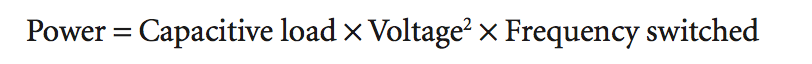Page 243: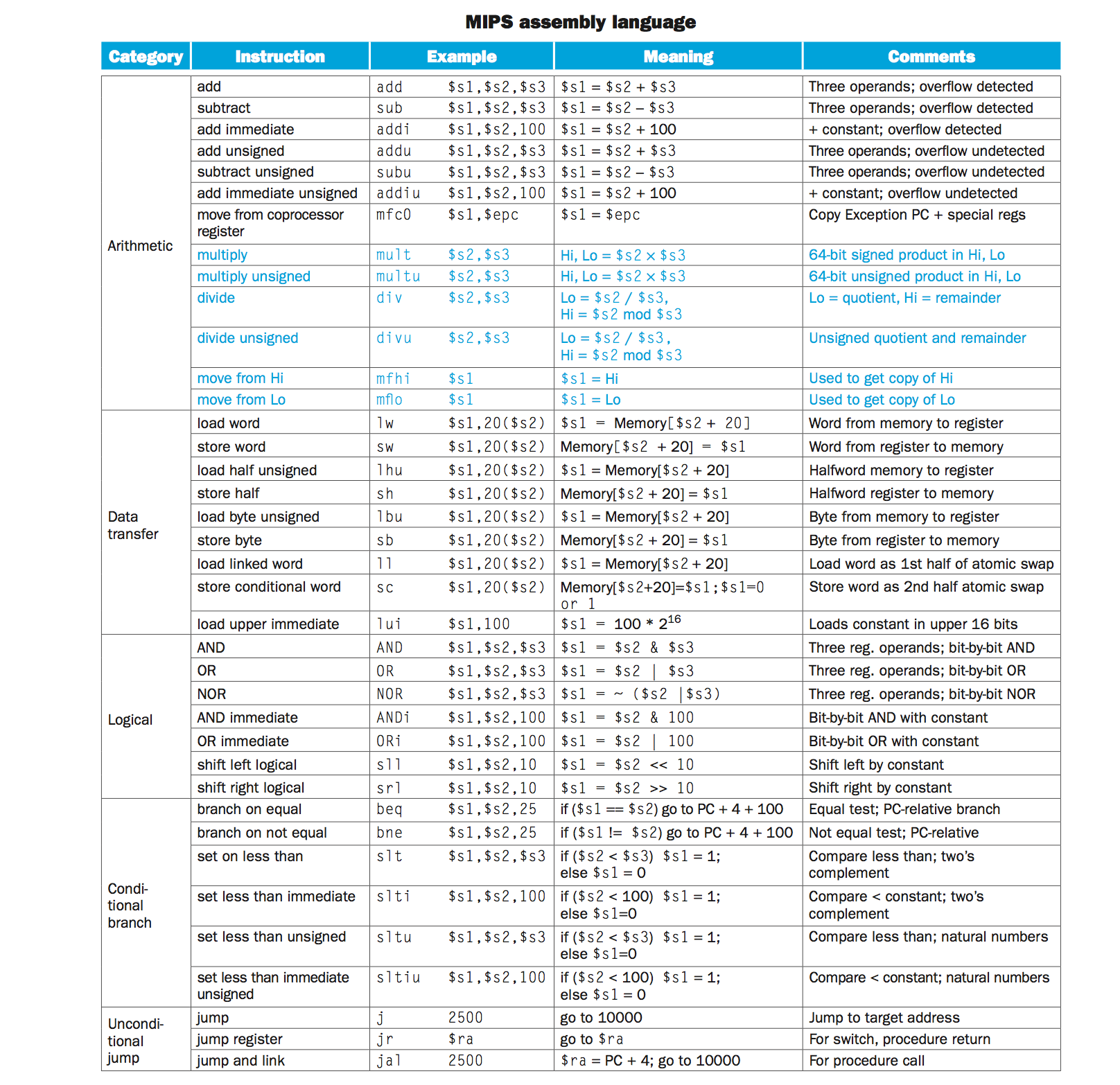Review floating point representation just pretty loosely.

op, rs, rt, rd, shamt, funct. Funct does the specific variant of the op code.

(r-type) for register. (i-type) for immediate.

Probably need to know the procedure call conditions: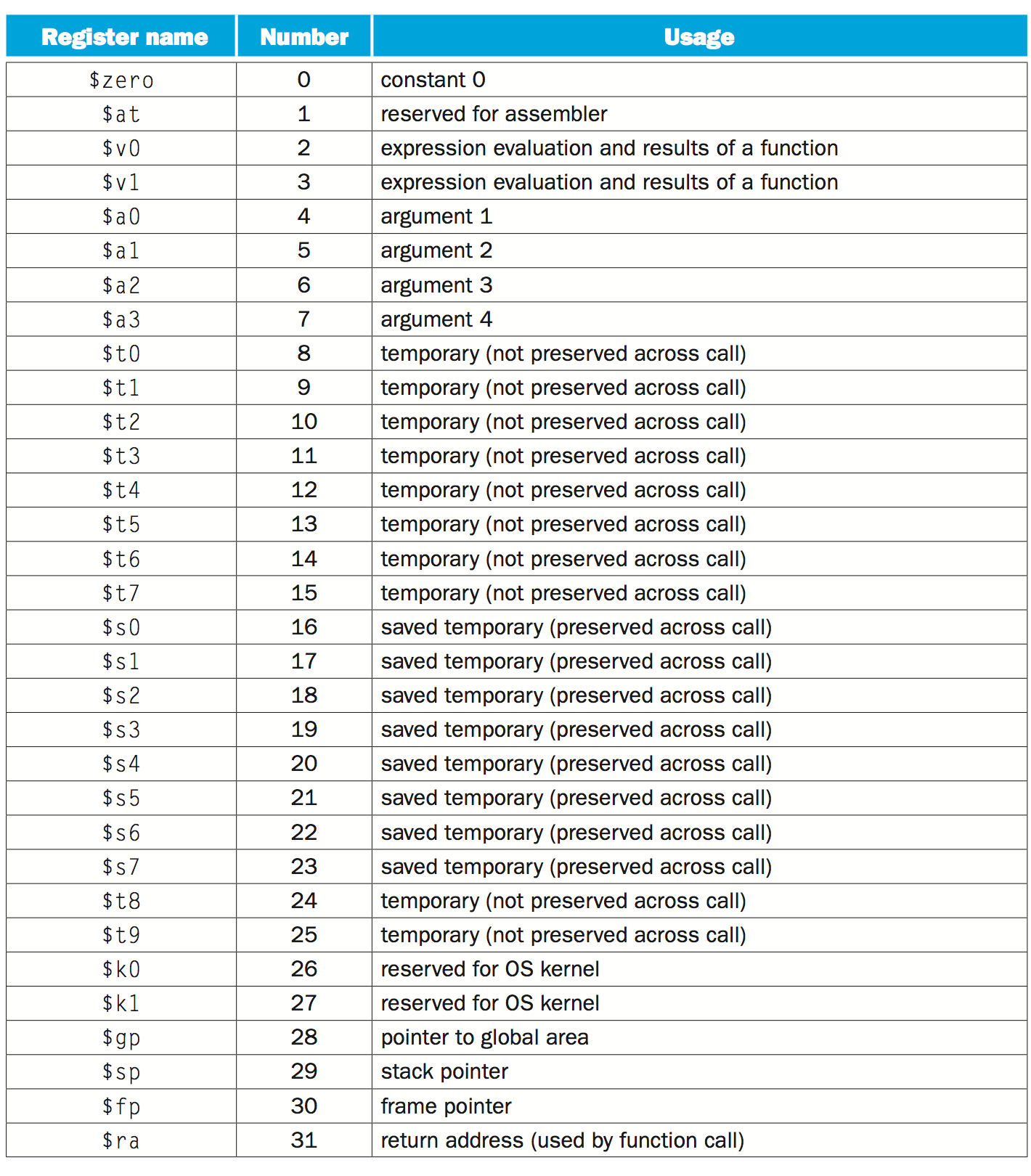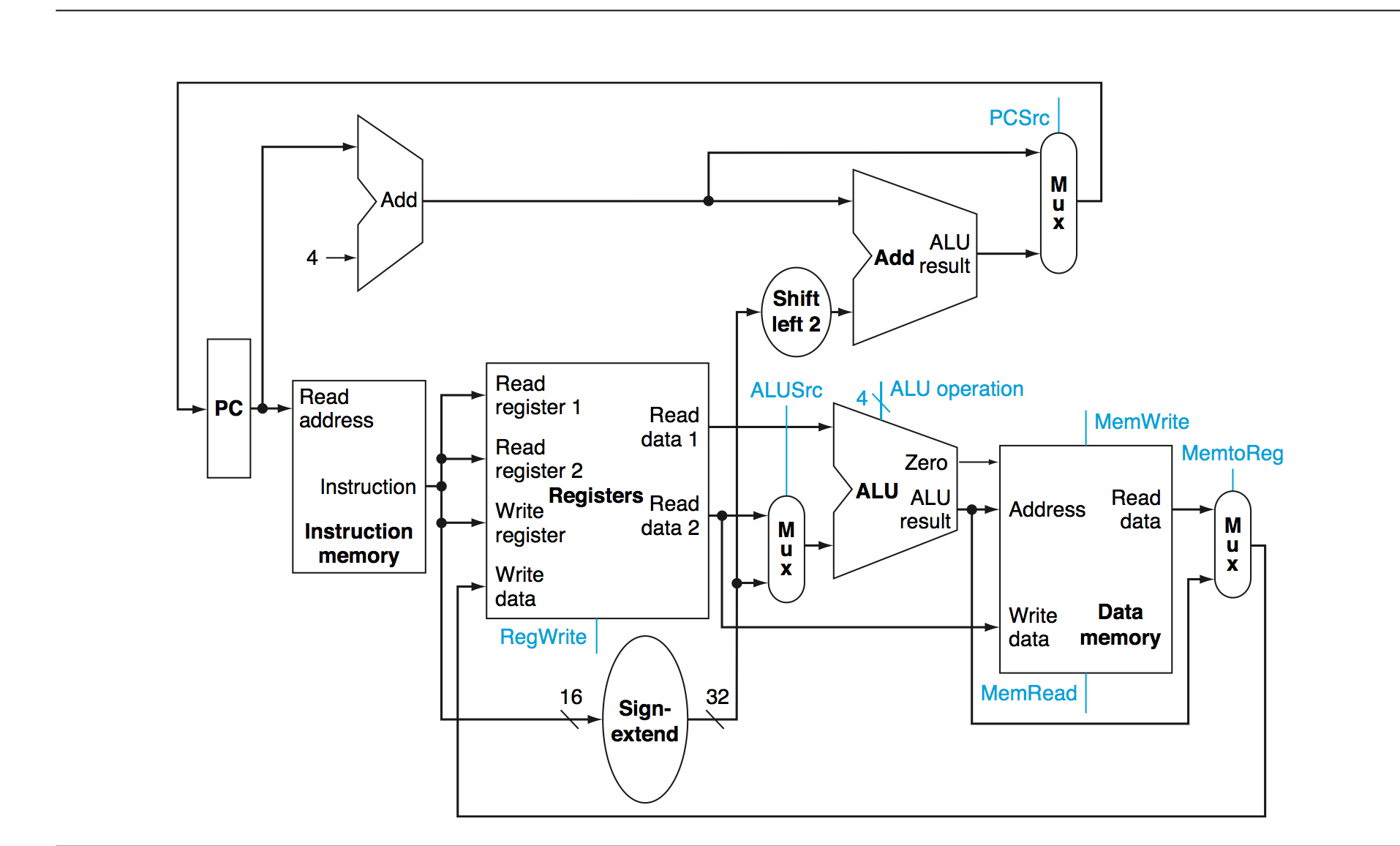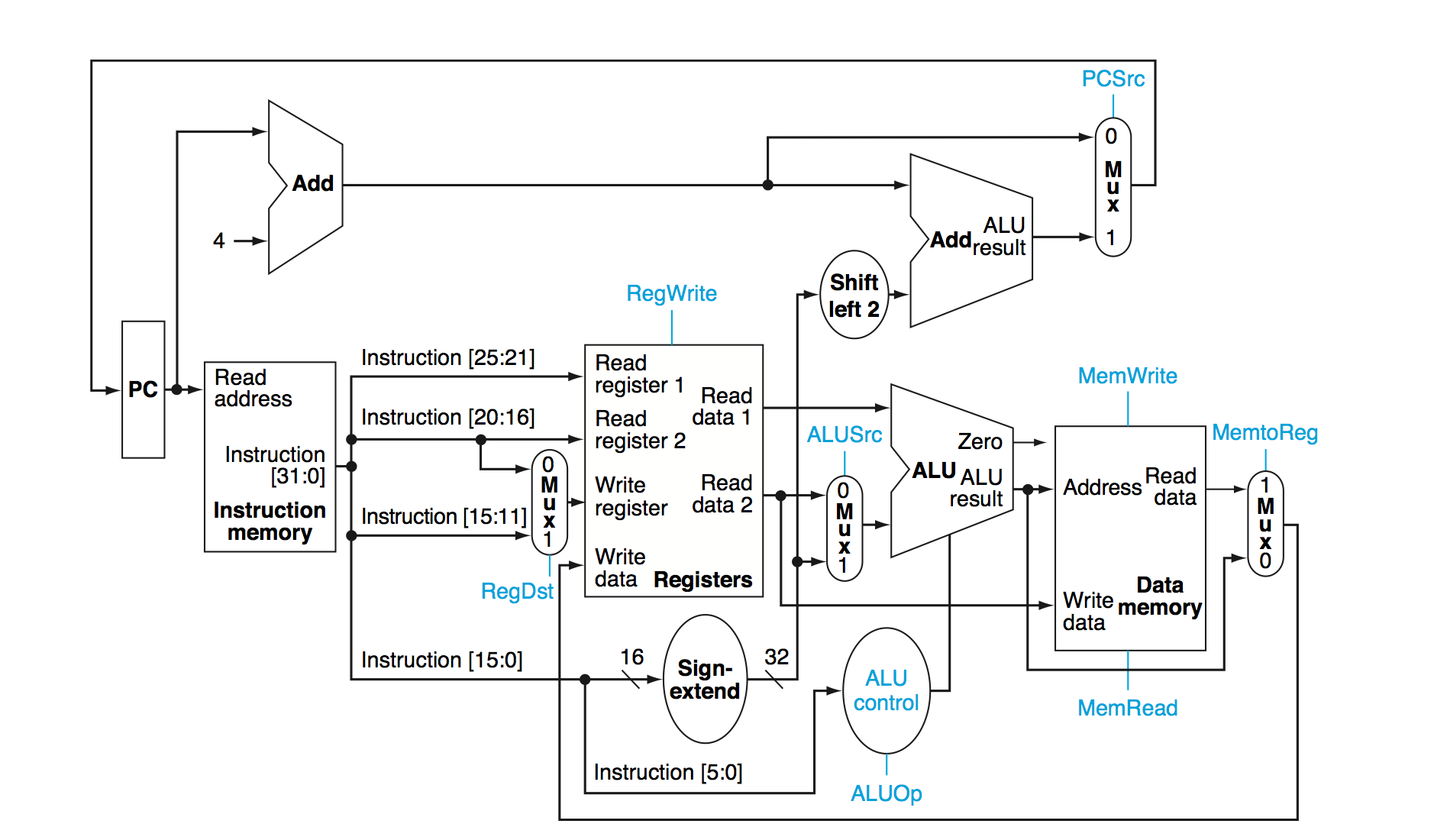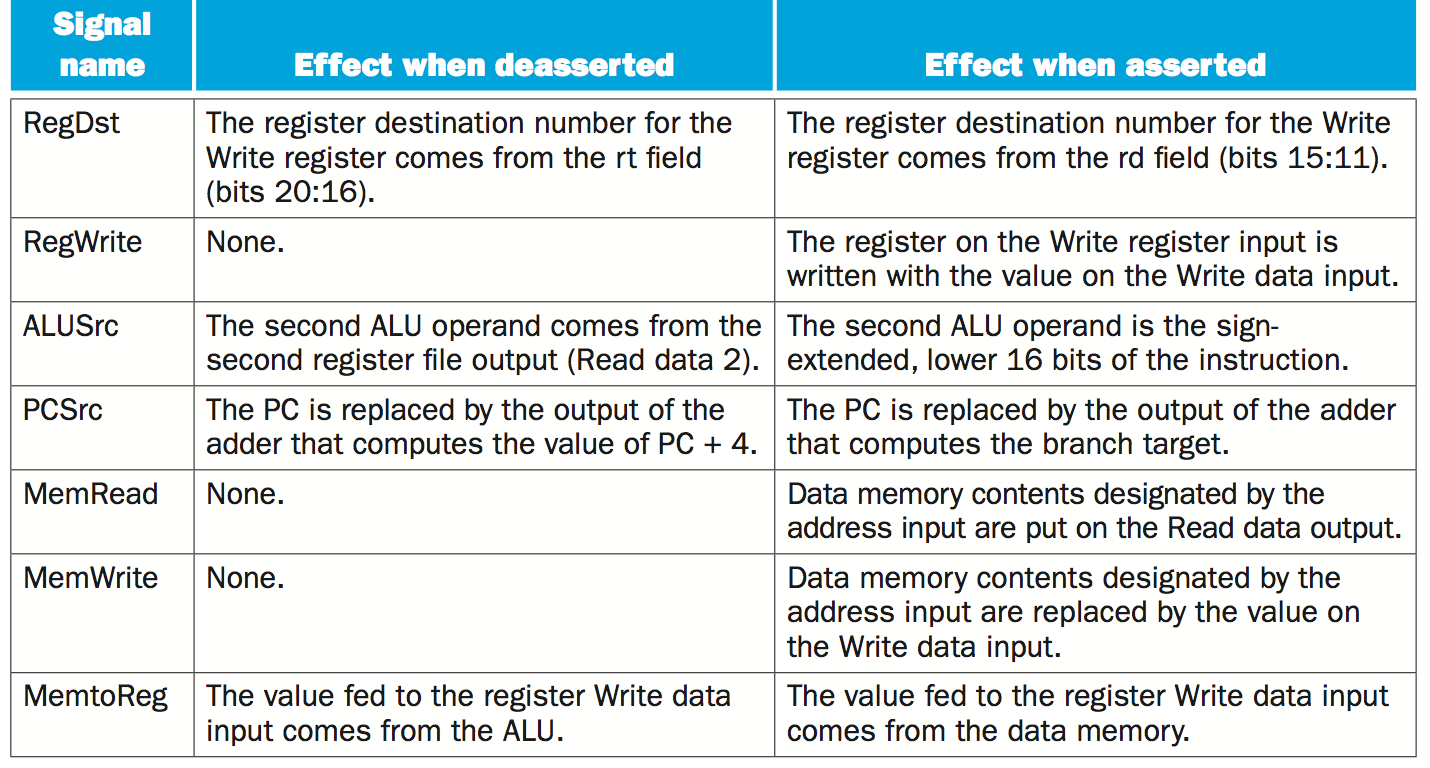Types of caches: Direct-mapped is where each memory location is mapped to exactly one location in the cache ( I think this would result in a cache that has two columns, one for the address and one for the value). These values (the keys) are called tags.

A “valid bit” is used to indicate whether what’s in that block of memory in the cache is actually usable (like left over from last startup/currently empty).

Need to understand SEXT and what it means.

No so a zero input into sext means you pad with 0s, and 1 input means you pad with sign…
So maybe it’s X unless it’s an immediate input, and then it’s a 1 if the number has the potential to be negative and 0 if the number must be positive?

For caches, we take the address that the data actually goes to, and take a section of it and use it as the cache address. How many bits we need depends on the size of the cache.

http://www.cs.umd.edu/class/sum2003/cmsc311/Notes/Memory/direct.html

You have fully associative and direct mapped cache. I think Direct Mapped looks one at a time while fully associative can find the right place by searching all simultaneously.

Say you have 32 bytes in each line, and 32K lines: low 5 bits determine which byte within line (offset). 15 bits to determine which of 32K lines has the data (index).

Things for cheat sheet:

Floating point formula
Maybe two amdahl’s law examples

Things I need to know refined:
Amdahl’s law
floating point (just write down formula)
Know how adder works: Really simple, you have a bunch of linked units that each have a CI (carry in), CO (carry out), and two inputs A and B, and then a 	result S. So each unit just figures out the sum of A+B+CI and then sets S and CO appropriately. To make this subtraction, all you have to do is XOR 	one of the inputs with a 1.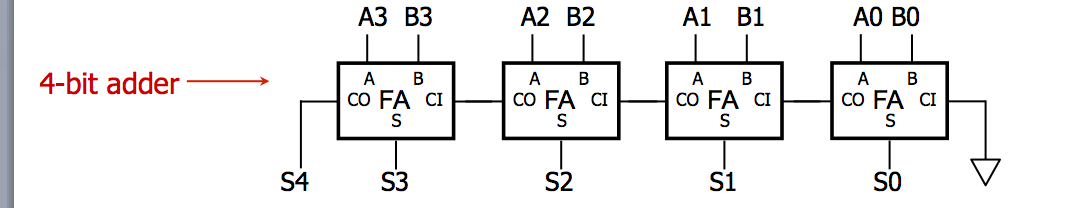Know how shifter works
A sequence of linked multiplexers where a control line (which is probably always a 1) is connected to all of the 1’s, and each input is connected to its 	multiplexer’s zero and also the 1 of the multiplexer above it. This would only allow shifting once to the left.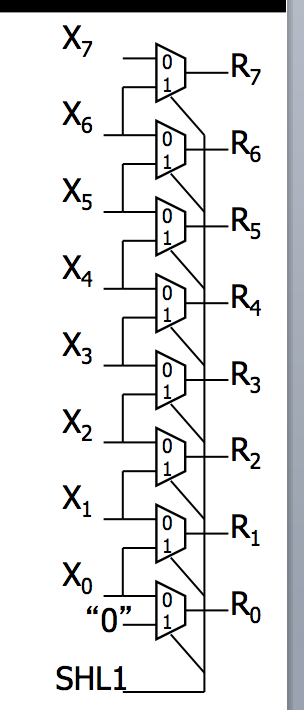How latches work (SRAM): There’s feedback here which allows the preservation of state if G is 0, and changing the state if G is 1.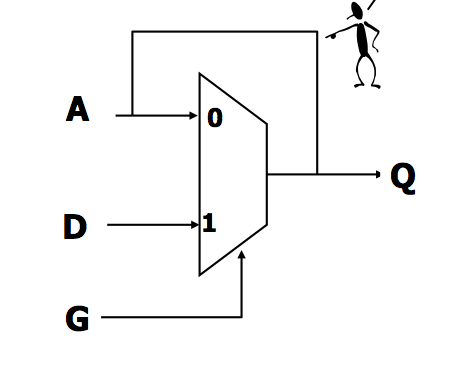How registers are constructed. Where EN is write-enabled, where the value of D is what would be written. and R is reset.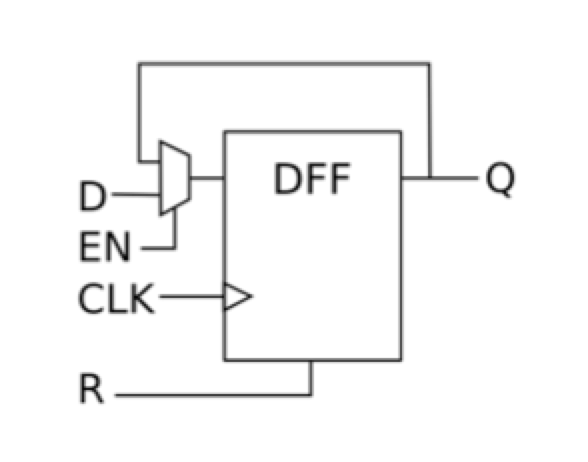How to design multiplexer.
Positive vs negative latch.
Caller/callee conventions.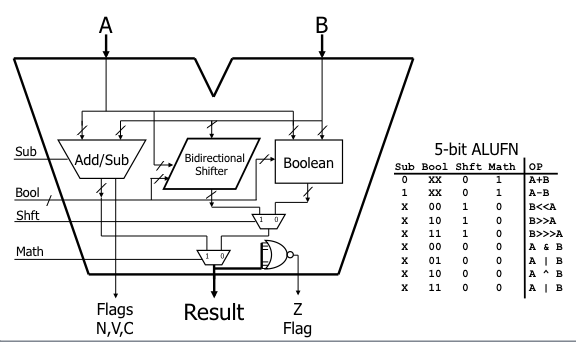Things I need to know:

2’s complement is: invert the digits given the number as positive and add 1.
Amdahl’s law: t_improved = t_affected / r_speedup + unaffected. “Suppose program runs in 100 seconds. Mltiplies are 80%. HOW MUCH IMPROVE RUN 4 TIMES FASTER. 25 = 80/R + 20. SO R = 16X.” How do we deal with this with regard to time?
Floating point: 1 sign bit, 8 exponent bits (2 to this power, offset is minus 127 so we have 2^(E-127)), 23 significant bits (this value is the actual value -1). N = -1^S(1+F)x2^(E-127).
Load Upper Immediate (LUI): loads the upper 16 bits of the register RT with a 16-bit immediate, and the rest with all zeros.
Maybe write down which registers are reserved for what. SP points to the top of the stack, the stack builds down. So subtract to add new value.
Triangle is ground, line is charge in transistor diagram.
SUM OF PRODUCTS. So for every output that’s a 1, make a gate that ORs all the inputs. How was problem 1 on the quiz done?
Know how adder works.
Know how shifter works.
Know how a ROM works. It’s like a sum of products. Only one of the wires can be high at a time.
Maybe have a diagram of how latches work (how things are remembered).
How is register constructed.
Understand fully associative: seems like you can put any value in any slot. When determining if you already have an address stored in the class, you can simultaneously do an XNOR on every tag in the cache to see if it’s stored. If you get a hit, check to see if that slot’s “valid” bit is 1 (must be 1 for a hit). You still have offset to keep track of what part of a cache line the value is actually stored.
Maybe review some MIPS code.
How to design a multiplexer (was on a pset)
Positive vs Negative latches?

Compiler -> assembler

CAN BRING TWO SIDED CHEAT SHEET.

Performance metrics:

Latency: How long it takes for a given one to go out.

throughput: How many can you get out .

Amdahl’s law: t_improved = t_affected / r_speedup + unaffected.

Suppose program runs in 100 seconds. Mltiplies are 80%. HOW MUCH IMPROVE RUN 4 TIMES FASTER. 25 = 80/R + 20. SO R = 16X.

Representing operands (characters, integers, non-integers: fixed and floating point). Know conversions binary, decimal, hex, two’s complement. How to encode positive integers. Between binary and decimal.

don’t worry about sign mag, NEED TO KNOW 2’S COMPLEMENT. Advantage of 2’s complement is that addition and subtraction are the same, so it’s easy.

Floating point format, most important is that you have 1 point something in the mantisa. So you assume a 1 (a binary 1).

You should know the assembly that we’ve done this semester.

Know how to use the load upper immediate instruction. Don’t need to know mul or div.

know the Addressing modes, absolute, indirect, and displacement.

Review pointers, they’ll have something on pointers.

Make sure we understand the stack. Remember how stack pointer works and which way it goes.

CMOS inverter. Remember how they work. Triangle is ground. Circle means that that connection is made.

Not necessarily be able to make gates, but be able to fill out the chart (super easy).

KNOW HOW TO DO SUM OF PRODUCTS. So for every output that’s a 1, make a gate that ORs all the inputs.

Know how multiplexer works.

KNOW HOW THE ADDER WORKS.

KNOW HOW SHIFTER WORKS

Know how a ROM works. It’s like a sum of products. Only one of the wires can be high at a time.

Remember how latches work (how things are remembered). Master and slave.

Synchronous systems: you can’t set the clock any shorter than the combinational logic that happens between two flip flops.

Could have a state machine.

Question on MIPS could be similar to what was in PSET 6.

How register is constructed.

Memory hiecharchy: Register, SRAM (cache), DRAM (RAM), Hard Disk.

UNDERSTAND Direct-mapped. UNDERSTAND FULLY ASSOCIATIVE.

Tag is the higher order bits. So you compare indexes first, then you compare tags to make sure that you’re getting the right address. Then you make the change. Make sure you get this.

5-stage pipeline. Understand the concepts. Need to understand hazards of pipelining. Put bubble in the pipeline.

Will have to be able to write assembly and C.
```